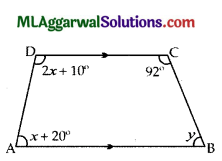ICSE Class 9 Maths Sample Question Paper 2 with Answers

Section – A
(Attempt all questions from this Section)

Question 1.
(a) If x – 3 – 2√2, find the value of x2 + -y.
Given =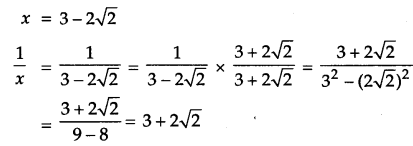(b) Factorize : 9x2 – 4 (y + 2x)2
9x2 -4(y + 2x)2 = (3x)2 – {2 (y + 2x)}2
= (3x)2 – (2y + 4x)2
= (3x + 2y + 4x) (3x – 2y – 4x)
= (7x + 2y) (-x -2y)
= – (x + 2y) (7x + 2y).(c) The area enclosed between the concentric circles is 770 cm2. Given that the radius of the outer circle is 21 cm, calculate the radius of the inner circle.
Given : The area enclosed between the circles = 770 cm2
Radius of outer circle (R) = 21 cm.
Let radius of inner circle be r.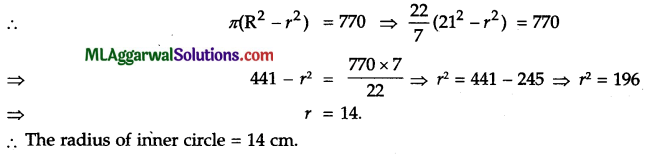Question 2.
(a) A man invests ₹46,875 at 4% p.a. compound interest for 3 years. Calculate :
(i) The interest for the first year.
(ii) The amount at the end of the second year.
(iii) The interest for the third year.(b) If ax = by = cz and b2 = ac, Prove that $$y=\frac{2 x z}{x+z}$$(c) In the following figure, AD and BC are equal perpendiculars to a line segment AB. Show that CD bisects AB.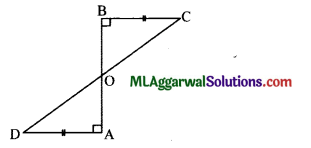Δ AOD =Δ BOC (Vertically opposite angles)
∴ ΔOAD ≅ ΔOBC (SAS axiom)
∴ OA = OB (c.p.c.t.)
Hence, CD bisects AB. Hence Proved.

Question 3.
(a) Solve the following equations by cross multiplication method :
3x – 7y = – 10, – 2x + y = 3.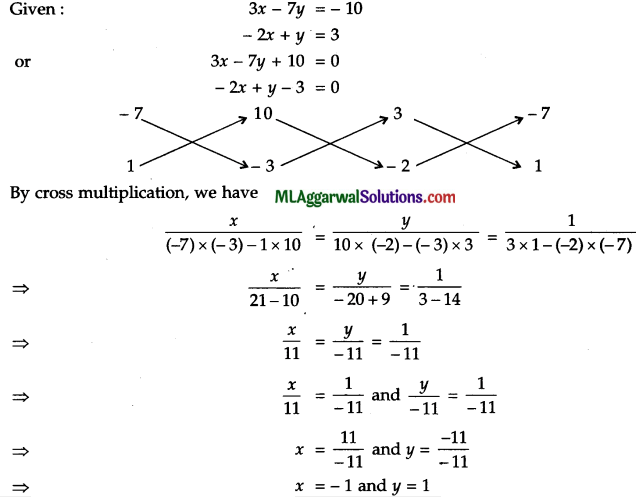(b) Find the value of :
2√2 cos 45° cos 60° + 2√3 sin 30° tan 60° – cos 0°
2√2 cos 45° cos 60° + 2√3 sin 30° tan 60° – cos 0°
$$=2 \sqrt{2} \times \frac{1}{\sqrt{2}} \times \frac{1}{2}+2 \sqrt{3} \times \frac{1}{2} \times \sqrt{3}-1$$

(c) Construct a frequency polygon for the following frequency distribution using a graph sheet.

 Marks 40 – 50 50-60 60 – 70 70-80 80 – 90 90 – 100 No. of Students 5 8 13 9 7 5

Use 1 cm – 10 marks and 1 cm = 5 students.Question 4.
(a) Express as a single logarithm :
2 log 3 – $$\frac{1}{2}$$ log 64 + log 16.
(b) If $$x+\frac{1}{x}=3$$,evaluate $$x^{3}+\frac{1}{x^{3}}$$
(c) Prove that the line joining mid-points of two parallel chords of a circle passes through the centre of the circle.(c) Given: AB || CD, M and N are mid-points of sides AB and CD respectively.
Construction: Join OM, ON and draw a straight line parallel to AB and CD.
Since, line segment joining the mid-point of the chord with centre of the circle is perpendicular to the chord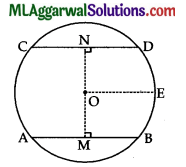∴ OM ⊥ AB and ON ⊥ CD
⇒ ∠AMO = 90° and ∠NOE = 90°
Now, ∠MOE = 90° (Co-interior angles, OE || AB)
∠NOE = 90° (Co-interior angles, OE || CD)
∠MOE + ∠NOF =90° + 900 = 1800
So, MON is a straight line passing through the centre of the circle.
Hence Proved.

Section – B
(Attempt any four questions from this Section)

Question 5.
(a) Find a point on the Y-axis which is equidistant from the points A (6, 5) and B (- 4, 3).
Given : A (6, 5), B (- 4, 3).
Let the point on the Y-axis be P (0, b).
According to the question,
AP = BP
⇒ AP2 = BP2
⇒ (6 – 0)2 + (5 – b)2 = (- 4 – 0)2 + (3 – b)2
⇒ 36 + 25 – 10b + V- = 16 + 9 – 6fo + b2
⇒ – 10b + 6b = 25 – 61
⇒  -4b =-36
⇒ b = 9
Required Point = (0,9)

(b) In the following figure, area of parallelogram ABCD is 29 cm2. Calculate the height of parallelogram ABEF, if AB = 5.8 cm.Area of parallelogram ABCD = 29 cm2, AB = 5.8 cm.
Area of parallelogram ABEF = 29 cm2 (area of parallelograms on same base are equal)
⇒ AB x Height = 29 cm2
⇒ $$\text { Height }=\frac{29 \mathrm{~cm}^{2}}{\mathrm{AB}}=\frac{29 \mathrm{~cm}^{2}}{5.8 \mathrm{~cm}}=5 \mathrm{~cm}$$

(c) A sum of money doubles itself at compound interest in 15 years. In how many years will it become eight times ?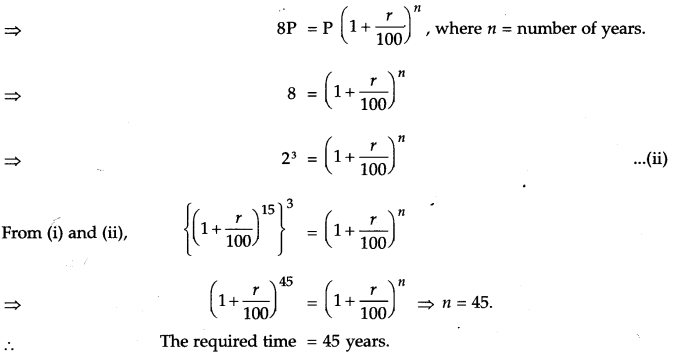Question 6.
(a) Construct the quadrilateral ABCD, given that AB = 5 cm, BC = 2.5 cm, CD = 6 cm,
∠BAD = 90° and the diagonal AC = 5.5 cm.
Given : AB = 5 cm, BC = 2.5 cm, CD = 6 cm, Z BAD 90°,
AC = 5.5 cm.
Steps of construction :
(1) Draw AB 5 cm.
(2) At A, draw ∠BAP = 90°.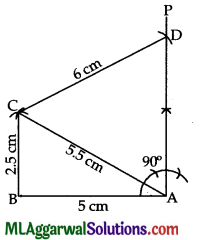(3) From B and A, draw arcs of lengths 2.5 cm and 5.5 cm, respectively which intersect at C.
(4) From C, cut-off AP at D such that CD = 6 cm.
Thus, ABCD is the required quadrilateral.

(b) Factorize : (a + 1) (a + 2) (a + 3) (a + 4) – 3.
(a + 1) (a + 2) (a + 3) (a + 4) – 3 = (a + 1) (a + 4) (a + 2) (a + 3) – 3
= (a2 + 5a + 4) (a2 + 5a + 6) – 3
= (p + 4) (p + 6) – 3  (Putting a2 + 5a = p)
= p2 + 6p + 4p + 24 – 3
= p2 + 10p + 21
= p2 + (7 + 3) p + 21
= p2 + 7p + 3p + 21
= p2 (p + 7) + 3 (p + 7) = (p + 7) (p + 3)
= (a2 + 5a + 7) (a2 + 5a + 3) (∵ p = a2 + 5a)

(c) In the following figure, D and E are mid-points of the sides AB and AC respectively. If BC = 6 cm and ∠B = 72°, compute (i) DE (ii) ∠ADE.Given : BC= 5.6 cm, ∠B = 72° and D, E are mid-points of sides AB, AC, respectively, (i)
The line joining the mid-points of any two sides of a triangle is parallel to the third side and is equal to half of it.Question 7.
(a) Evaluate without using tables :
$$\left(\frac{\cos 47^{\circ}}{\sin 43^{\circ}}\right)^{2}+\left(\frac{\sin 72^{\circ}}{\cos 18^{\circ}}\right)^{2}-2 \cos ^{2} 45^{\circ}$$(b) Solve:
$$\left(\sqrt{\frac{3}{5}}\right)^{x+1}=\frac{125}{27}$$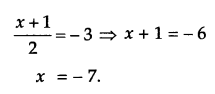(c) In ΔABC, ∠ACB = 90°, AB = c unit, BC = a unit,
AC = b unit, C perpendicular to AB and CD = p unit.
Prove that $$\frac{1}{p^{2}}=\frac{1}{a^{2}}+\frac{1}{b^{2}}$$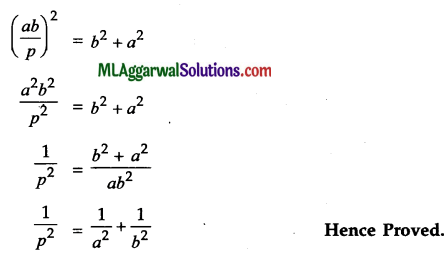Question 8.
(a) If $$x^{2}+\frac{1}{x^{2}}=27$$,find the values of :
(i) $$x+\frac{1}{x}$$
(ii) $$x-\frac{1}{x}$$
(iii) $$x^{2}-\frac{1}{x^{2}}$$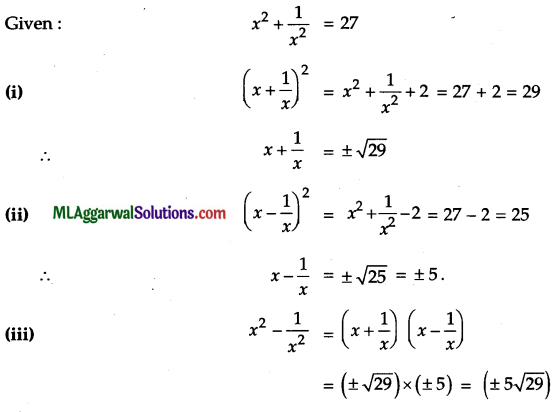(b) If 1 is added to the numerator of a fraction, it becomes $$\frac{1}{5}$$. If 1 is subtracted from the denominator, it becomes $$\frac{1}{7}$$. Find the fraction.(c) Find the mean and median of the numbers :
41, 39, 52, 48, 54, 62, 46, 52, 40, 96, 42, 40, 98, 60, 52.
41, 39, 52, 48, 54, 62, 46, 52, 40, %, 42, 40, 98, 60, 52.
∴ ∑ x =822, n=15
∴ $$\text { Mean }=\frac{\sum x}{n}=\frac{822}{15}=54.8$$
Rearranging in ascending order, we get
39, 40, 40, 41, 42, 46, 48, 52, 52, 52, 54, 60, 62, 96, 98Question 9.
(a) The volume of a cuboidal block of silver is 10368 cm3. If its dimensions are in the ratio 3:2:1, find :
(i) Dimensions of the block.
(ii) Cost of gold polishing its entire surface at ₹0.50 per cm2.
(a) (i) Ratio of dimensions = 3:2:1
Let its length, breadth and height be 3x cm, 2x cm and x cm respectively.
Volume of block = 3× x 2x × x = 10368
⇒ 6x3 = 10368
$$x^{3}=\frac{10368}{6}=1728 \Rightarrow x=12$$
Length (l) =3x = 3 × 12=36cm
Breadth (b) = 2x = 2 × 12=24cm
Height (h) =x = 12 cm(ii) Total surface area =2(lb+lh+bh)=2(36 x 24+36 x 12+24x 12)
= 2 (864 + 432 + 288)
= 2 x 1584 = 3168 cm2
∵ Rate of gold polishing = ₹ 0.50 = ₹$$\frac{1}{2}$$
Total cost of gold polishing of entire surface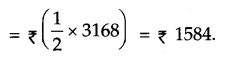(b) Factorize : 2 – y (7 – 5y).
2 – y (7 – 5y) = 2-7y + 5y2
= 2 – (5 + 2) y + 5y2 = 2 – 5y – 2y + 5y2
= 1 (2 – 5y) – y (2 – 5y) = (2 – 5y) (1 – y)

(c) Solve graphically : x – 2y = 1; x + y – 4.
x – 2y =1 ……….(i)
x + y = 4 ……………(ii)
from (i)∴ The points are (1, 0), (3, 1), (5, 2)

From (ii)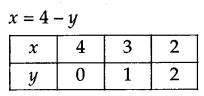The points are (4, 0), (3, 1), (2, 2)
These points are plotted on the graph.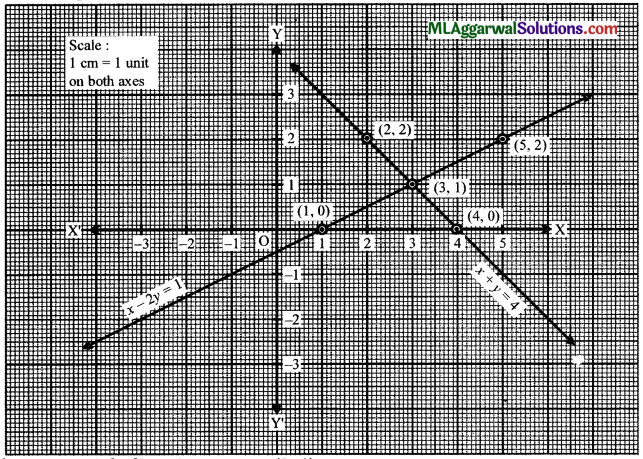The two straight lines intersect at (3, 1)
∴ x=3,y=1Question 10.
(a) From the adjoining figure, find the values of :
(i) cot2 x – cosec2 x
(ii) $$\tan ^{2} y-\frac{1}{\cos ^{2} y}$$
(a) In AABD,
AB2 = AD2 + BD2 (By Pythagoras theorem)
= 42 + 32 = 16 + 9 = 25
AB = √25 = 5.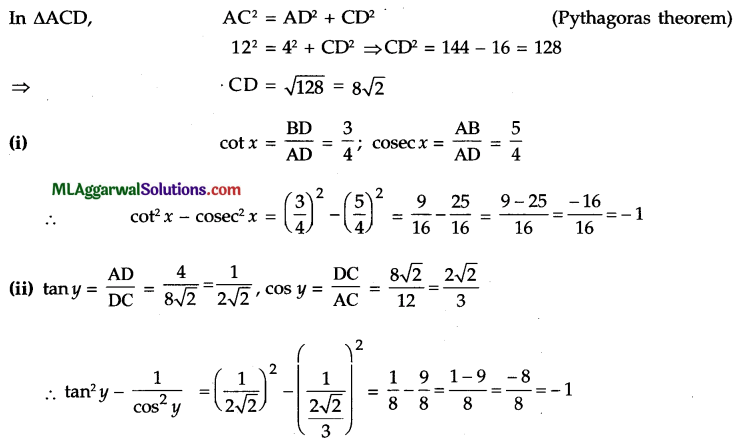(b) $$\text { If } \frac{\log a}{b-c}=\frac{\log b}{c-a}=\frac{\log c}{a-b}, \text { prove that }: a^{a} . b^{b} \cdot c^{c}=1 \text { . }$$
$$\frac{\log a}{b-c}=\frac{\log b}{c-a}=\frac{\log c}{a-b}=k(\text { say })$$
log a =k(b – c); log b = k(c-a); log c = k(a-b)
Now, a log a + b log c + c log c = ak (b – c) + bk (c – a) + ck (a – b)
⇒ log ab + log bb + log cc = kab – kac + kbc – kab + kac – kbc
⇒ log (aa. bb . cc) = 0
⇒ log (aa. bb . cc)= log 1
⇒ aa. bb. cc = 1.
Hence Proved.

(c) Prove that √2 is not a rational number.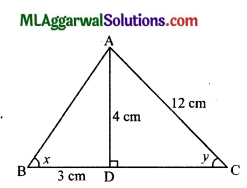Let us assume that√2 is a rational number.
If $$\sqrt{2}=\frac{p}{q}, p, q \in \mathrm{I}$$ have no comman factor and C”q≠ 0.
$$2=\frac{p^{2}}{q^{2}} \Rightarrow p^{2}=2 q^{2} \Rightarrow p^{2}$$ C”is an even integer
⇒ p is an even integer
⇒ p = 2m, where m∈I
⇒ p2 = 4m2 ⇒ 2y2 = 4m2 ⇒ q2 = 2m2
⇒ q2 is an even integer
⇒ y is an even integer.
Thus, p and q are both even integers and therefore, have a common factor 2 which contradicts that p and q have no common factor.
√2 is not a rational number.
Hence Proved.Question 11.
(a) Simplify: $$\left(a+\frac{1}{a}\right)^{2}-\left(a-\frac{1}{a}\right)^{2}$$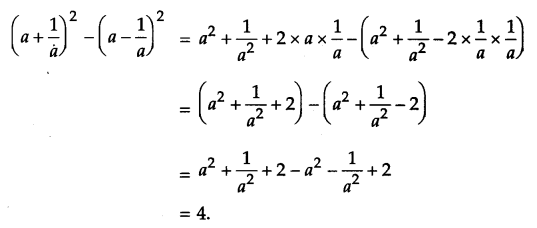(c) Simplify: $$\frac{(25)^{3 / 2} \times(243)^{3 / 5}}{(16)^{5 / 4} \times(8)^{4 / 3}}$$